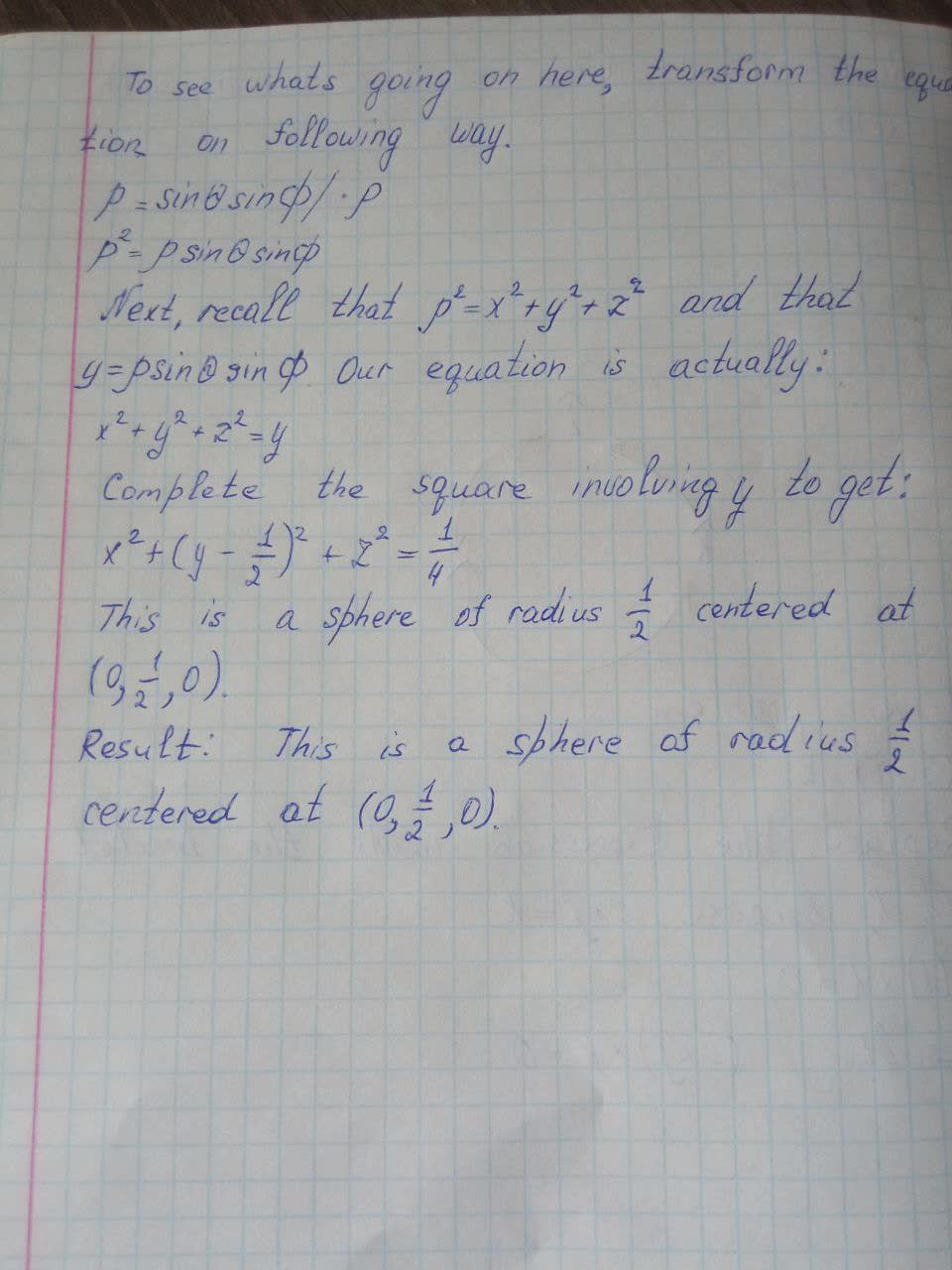Question# Identify the surface whose equation is given. p=\sin\theta\sin\phi

Trigonometric Functions
ANSWEREDIdentify the surface whose equation is given. $$p=\sin\theta\sin\phi$$